Integral of ln²(x)

In this post I will show you how to find the anti-derivative of the function f(x)=ln²(x).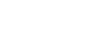We are going to use integration by parts: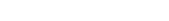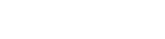Now we can write u times v minus the integral of v times du: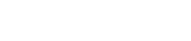Let’s bring out the 2 (linearity); also, the two x‘s will cancel out.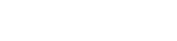We saw here that the integral of ln(x) is equal to xln(x)-x, therefore: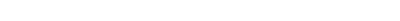If you want, you can factor out the x: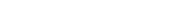Finally,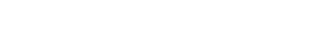That’s it!

If you liked this post give it a like and if you are interested in more integrals and derivatives, subscribe to receive notifications!

Join 31 other subscribers.